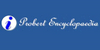### DirectrixIn mathematics, a directrix is a straight line associated to a geometric object, such as: ==Other uses== ...
Found on http://en.wikipedia.org/wiki/Directrix

### Directrix• (n.) A line along which a point in another line moves, or which in any way governs the motion of the point and determines the position of the curve generated by it; the line along which the generatrix moves in generating a surface. • (n.) A directress. • (n.) A straight line so situated with respect to a conic section that the dist...
Found on http://thinkexist.com/dictionary/meaning/directrix/

### directrix(from the article `cone`) ...the surface traced by a moving straight line (the generatrix) that always passes through a fixed point (the vertex). The path, to be definite, is ... ...that is traced by a straight line (the generatrix) that always moves parallel to itself or some fixed line or direction (the axis). The path, to ... ...b...
Found on http://www.britannica.com/eb/a-z/d/54

### directrix1. A directress. ... 2. <geometry> A line along which a point in another line moves, or which in any way governs the motion of the point and determines the position of the curve generated by it; the line along which the generatrix moves in generating a surface. A straight line so situated with respect to a conic section that the distance of a...
Found on http://www.encyclo.co.uk/local/20973

### DirectrixDi·rect'rix noun ; plural English Directrixes Latin Directrices 1. A directress. [ R.] Jer. Taylor. 2. (Geom.) (a) A line along which a point in another line moves, or which in any way governs the motion of the point and determines the posi...
Found on http://www.encyclo.co.uk/webster/D/72

### DirectrixIn mathematics, a directrix is a line perpendicular to the axis of a conic section, and so placed that the distance from it of any point in the curve is to the distance of the same point from the focus in a constant ratio. The name is also given to any line, whether straight or not, that is required for the description of a curve. The directrix of ...
Found on http://www.probertencyclopaedia.com/browse/GD.HTM

### directrixThe line that, together with a point called the focus, serves to define a conic section as the locus of points whose distance from the focus is proportional to the horizontal distance from the directrix. If the ratio r = 1, the conic is a parabola, if r < 1, it is an ellipse, and if r > 1, it is a h...
Found on http://www.daviddarling.info/encyclopedia/D/directrix.html
No exact match found